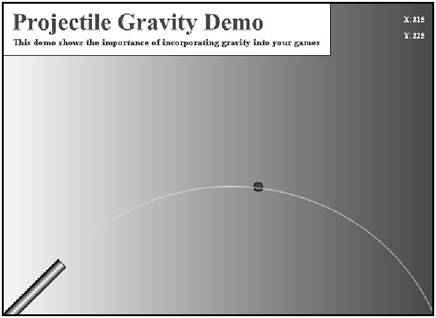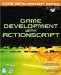# Working with Gravity

 [ LiB ]

Gravity is a downward-accelerating force of 9.8 m/s/s. There is no need to program at this rate if your game world is not measured on the same scale as the Earth's gravitational pull. So what should you do? You eyeball it. You can only model so much within a game.

To make things more interesting, projectiles move in a trajectory. The reason this happens is because projectiles on earth are constantly affected by gravitational pull, which causes trajectories to be parabolic arcs , meaning they follow the general shape of a parabola as they travel up and back down. If this wasn't so, they would fly in a linear path and never come back down. These trajectories can be accurately replicated with complicated formulas, but they can also be replicated with some slick programming techniques.

See Figure 12.6 to see how the projectile in the next demo moves on a path.

##### Figure 12.6. A trajectory pathIf you open up GDA_PROG12.3.fla, you'll notice a small cannon on the left side of the screen. If you test the movie, you'll see that the cannon fires a projectile across the screen. When the projectile lands, the cannon fires again. One might think that a lot of difficult math went into this, but there's nothing difficult about it. Check out the code that I embedded into that projectile:

` // Game Development with ActionScript // By Lewis Moronta (c) 2003 // This program demonstrates the // use of gravity in a game. onClipEvent(load) {   // Initialize some properties   xv =  10;   yv = -10;   gravity = .5; } onClipEvent(enterFrame) {   // Add some motion   _x += xv;   _y += yv;   // Add some gravity   yv += gravity;   // Reset if off the screen   if (_y > 400) {     _x = 65;     _y = 324;     yv = -10;   } } `

The first thing I did when the Movie Clip loaded was to create a few new properties. These properties will control the projectile's flow through the air.

` onClipEvent(load) {   // Initialize some properties   xv =  10;   yv = -10;   gravity = .5; } `

I initialized two velocity variables . They lead the projectile up and to the right. The gravity variable will be used to pull the projectile downit will directly affect the yv velocity variable. Let's see how in the next part of the code.

Besides creating an onClipEvent(enterFrame) handler, I also set up the program so velocity is always being applied to the projectile, even when it is at rest.

` onClipEvent(enterFrame) {   // Add some motion   _x += xv;   _y += yv; `

As we have a y velocity variable, which is vertical, gravity should affect the variable if you want realistic-looking results. This is why I affect the velocity variable on every frame that passes with the following line:

` // Add some gravity   yv += gravity; `

I finally took the liberty of performing a reset on all of the program variables once the ball was off the screen. This will cause the cannon to repeat and fire.

` // Reset if off the screen   if (_y > 400) {     _x = 65;     _y = 324;     yv = -10; } `

And you thought you were going to learn about boring theories without applications.

 [ LiB ]Game Development with ActionScript
ISBN: 1592001106
EAN: 2147483647
Year: 2004
Pages: 162
Authors: Lewis Moronta